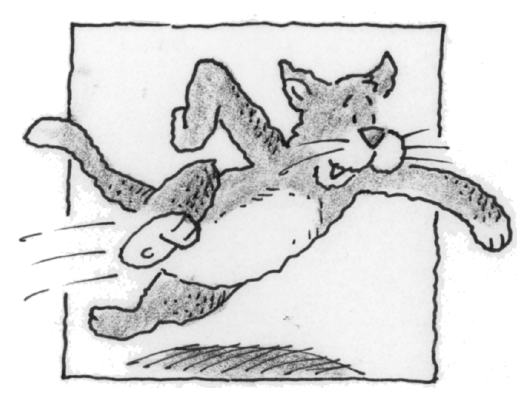### Home > APCALC > Chapter 6 > Lesson 6.5.1 > Problem6-171

6-171.

Bob’s cat, Alice, is still doing wind sprints up and down his hallway. This time suppose that Alice’s acceleration after $t$ seconds is given by the equation $a(t) = −π \sin( \frac { \pi t } { 5 } )$. As Alice sprints down the hallway, her initial velocity is $6$ feet per second, so $v(0) = 6$.

1. Write an equation for Alice’s velocity at time $t$.

To write the velocity formula, use the given initial velocity.

2. Calculate Alice’s displacement between $t = 5$ and $t = 10$.

$\text{displacement }=\int_{t=a}^{t=b}\text{(velocity)}dt$

$\text{total distance }=\int_{t=a}^{t=b}\text{(speed)}dt=\int_{t=a}^{t=b}\left | \text{velocity} \right |dt$